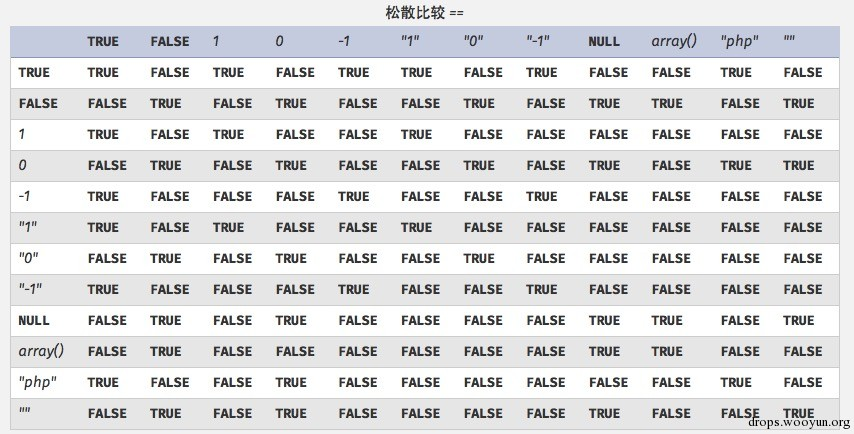2年前 (2018-10-18) 网友投稿

[收起] 文章目录

# 0x00 弱类型初探

```
#!php
<?php
\$var = 1;
\$var = array();
\$var = "string";
?>
```

php不会严格检验传入的变量类型，也可以将变量自由的转换类型。

\$a = null; \$b = false; //为真

\$a = ''; \$b = 0; //同样为真

# 0x02 知识预备 php内核之zval结构

zval的定义在zend/zend.h

```
#!c
typedef struct _zval_struct zval;

struct _zval_struct {
/* Variable information */
zvalue_value value;     /* value */
zend_uint refcount__gc;
zend_uchar type;    /* active type */
zend_uchar is_ref__gc;
};

typedef union _zvalue_value {
long lval;  /* long value */
double dval;    /* double value */
struct {
char *val;
int len;
} str;
HashTable *ht;  /* hash table value */
zend_object_value obj;
} zvalue_value;
```

# 0x03变量的强制转换

## 当int遇上string

##### cp.1 数学运算

```
#!php
var_dump(0 == '0'); // true
var_dump(0 == 'abcdefg'); // true
var_dump(0 === 'abcdefg'); // false
var_dump(1 == '1abcdef'); // true
```

intval再和整数部分比较,其实也就是改变了zval.type的内容 尤为注意的是，'1assd'的转换后的值是1，而‘asdaf’是0

```
#!php
var_dump(intval('3389a'));//输出3389
```

```
#!php
if(\$a>1000){
mysql_query('update ... .... set value=\$a')
}
```

#### cp.2 语句条件的松散判断

php的switch使用了松散比较. \$which会被自动intval变成0

```
#!php
<?php
if (isset(\$_GET['which']))
{
\$which = \$_GET['which'];
switch (\$which)
{
case 0:
case 1:
case 2:
require_once \$which.'.php';
break;
default:
echo GWF_HTML::error('PHP-0817', 'Hacker NoNoNo!', false);
break;
}
```

#### cp.3 函数的松散判断

```
#!php
var_dump(in_array("abc", \$array));
```

in_array — 检查数组中是否存在某个值 参数

needle 待搜索的值。

Note: 如果 needle 是字符串，则比较是区分大小写的。 haystack 这个数组。

strict 如果第三个参数 strict 的值为 TRUE 则 in_array() 函数还会检查 needle 的类型是否和 haystack 中的相同。

```
#!php
var_dump(in_array("abc", \$array1));</br>
var_dump(in_array("1bc", \$array2));
```

array_search也是一样的原理

```
#!php
\$a = 'asdfgh'；//字符串类型的a</br>
echo \$a；  //根据php的offset 会输出'd'</br>
echo \$a[x]；  //根据php的预测，这里应该是int型，那么输入string，就会被intval成为0 也就是输出'a'
```

### 当数组遇上string

Array转换整型int/浮点型float会返回元素个数；

```
#!php
if(!strcmp(\$c,\$d) && \$c!==\$d){
...
}
```

strcmp() 函数比较两个字符串。

```
0 - 如果两个字符串相等
<0 - 如果 string1 小于 string2
>0 - 如果 string1 大于 string2
```

```
#!php
http://localhost:8888/1.php?a[]=1
var_dump(strcmp(\$_GET[a],'a'));
```# 0x04时时防备弱类型

\$array =array();的习惯。

//下面这个css和插件后台设置的主题有关系，如果需要换样式，则需要修改以下CSS名称### 注册